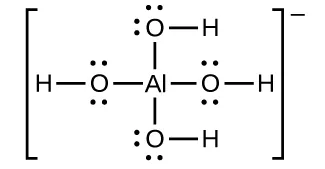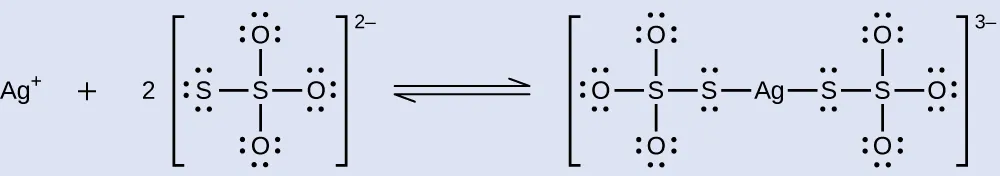Chemistry: Atoms First 2e

# 15.3Coupled Equilibria

Chemistry: Atoms First 2e15.3 Coupled Equilibria

### Learning Objectives

By the end of this section, you will be able to:

• Describe examples of systems involving two (or more) coupled chemical equilibria
• Calculate reactant and product concentrations for coupled equilibrium systems

As discussed in preceding chapters on equilibrium, coupled equilibria involve two or more separate chemical reactions that share one or more reactants or products. This section of this chapter will address solubility equilibria coupled with acid-base and complex-formation reactions.

An environmentally relevant example illustrating the coupling of solubility and acid-base equilibria is the impact of ocean acidification on the health of the ocean’s coral reefs. These reefs are built upon skeletons of sparingly soluble calcium carbonate excreted by colonies of corals (small marine invertebrates). The relevant dissolution equilibrium is

$CaCO3(s)⇌Ca2+(aq)+CO3−2(aq)Ksp=8.7×10−9CaCO3(s)⇌Ca2+(aq)+CO3−2(aq)Ksp=8.7×10−9$

Rising concentrations of atmospheric carbon dioxide contribute to an increased acidity of ocean waters due to the dissolution, hydrolysis, and acid ionization of carbon dioxide:

$CO2(g)⇌CO2(aq)CO2(g)⇌CO2(aq)$
$CO2(aq)+H2O(l)⇌H2CO3(aq)CO2(aq)+H2O(l)⇌H2CO3(aq)$

Inspection of these equilibria shows the carbonate ion is involved in the calcium carbonate dissolution and the acid hydrolysis of bicarbonate ion. Combining the dissolution equation with the reverse of the acid hydrolysis equation yields

The equilibrium constant for this net reaction is much greater than the Ksp for calcium carbonate, indicating its solubility is markedly increased in acidic solutions. As rising carbon dioxide levels in the atmosphere increase the acidity of ocean waters, the calcium carbonate skeletons of coral reefs become more prone to dissolution and subsequently less healthy (Figure 15.7).

Figure 15.7 Healthy coral reefs (a) support a dense and diverse array of sea life across the ocean food chain. But when coral are unable to adequately build and maintain their calcium carbonate skeletons because of excess ocean acidification, the unhealthy reef (b) is only capable of hosting a small fraction of the species as before, and the local food chain starts to collapse. (credit a: modification of work by NOAA Photo Library; credit b: modification of work by “prilfish”/Flickr)

The dramatic increase in solubility with increasing acidity described above for calcium carbonate is typical of salts containing basic anions (e.g., carbonate, fluoride, hydroxide, sulfide). Another familiar example is the formation of dental cavities in tooth enamel. The major mineral component of enamel is calcium hydroxyapatite (Figure 15.8), a sparingly soluble ionic compound whose dissolution equilibrium is

$Ca5(PO4)3OH(s)⇌5Ca2+(aq)+3PO43−(aq)+OH−(aq)Ca5(PO4)3OH(s)⇌5Ca2+(aq)+3PO43−(aq)+OH−(aq)$
Figure 15.8 Crystal of the mineral hydroxyapatite, Ca5(PO4)3OH, is shown here. The pure compound is white, but like many other minerals, this sample is colored because of the presence of impurities.

This compound dissolved to yield two different basic ions: triprotic phosphate ions

$PO43−(aq)+H3O+(aq)⟶H2PO42−(aq)+H2O(l)PO43−(aq)+H3O+(aq)⟶H2PO42−(aq)+H2O(l)$
$H2PO42−(aq)+H3O+(aq)⟶H2PO4−(aq)+H2O(l)H2PO42−(aq)+H3O+(aq)⟶H2PO4−(aq)+H2O(l)$
$H2PO4−(aq)+H3O+(aq)⟶H3PO4(aq)+H2O(l)H2PO4−(aq)+H3O+(aq)⟶H3PO4(aq)+H2O(l)$

and monoprotic hydroxide ions:

$OH−(aq)+H3O+⟶2H2OOH−(aq)+H3O+⟶2H2O$

Of the two basic productions, the hydroxide is, of course, by far the stronger base (it’s the strongest base that can exist in aqueous solution), and so it is the dominant factor providing the compound an acid-dependent solubility. Dental cavities form when the acid waste of bacteria growing on the surface of teeth hastens the dissolution of tooth enamel by reacting completely with the strong base hydroxide, shifting the hydroxyapatite solubility equilibrium to the right. Some toothpastes and mouth rinses contain added NaF or SnF2 that make enamel more acid resistant by replacing the strong base hydroxide with the weak base fluoride:

$NaF+Ca5(PO4)3OH⇌Ca5(PO4)3F+Na++OH−NaF+Ca5(PO4)3OH⇌Ca5(PO4)3F+Na++OH−$

The weak base fluoride ion reacts only partially with the bacterial acid waste, resulting in a less extensive shift in the solubility equilibrium and an increased resistance to acid dissolution. See the Chemistry in Everyday Life feature on the role of fluoride in preventing tooth decay for more information.

### Chemistry in Everyday Life

#### Role of Fluoride in Preventing Tooth Decay

As we saw previously, fluoride ions help protect our teeth by reacting with hydroxylapatite to form fluorapatite, Ca5(PO4)3F. Since it lacks a hydroxide ion, fluorapatite is more resistant to attacks by acids in our mouths and is thus less soluble, protecting our teeth. Scientists discovered that naturally fluorinated water could be beneficial to your teeth, and so it became common practice to add fluoride to drinking water. Toothpastes and mouthwashes also contain amounts of fluoride (Figure 15.9).

Figure 15.9 Fluoride, found in many toothpastes, helps prevent tooth decay (credit: Kerry Ceszyk).

Unfortunately, excess fluoride can negate its advantages. Natural sources of drinking water in various parts of the world have varying concentrations of fluoride, and places where that concentration is high are prone to certain health risks when there is no other source of drinking water. The most serious side effect of excess fluoride is the bone disease, skeletal fluorosis. When excess fluoride is in the body, it can cause the joints to stiffen and the bones to thicken. It can severely impact mobility and can negatively affect the thyroid gland. Skeletal fluorosis is a condition that over 2.7 million people suffer from across the world. So while fluoride can protect our teeth from decay, the US Environmental Protection Agency sets a maximum level of 4 ppm (4 mg/L) of fluoride in drinking water in the US. Fluoride levels in water are not regulated in all countries, so fluorosis is a problem in areas with high levels of fluoride in the groundwater.

The solubility of ionic compounds may also be increased when dissolution is coupled to the formation of a complex ion. For example, aluminum hydroxide dissolves in a solution of sodium hydroxide or another strong base because of the formation of the complex ion $Al(OH)4−.Al(OH)4−.$The equations for the dissolution of aluminum hydroxide, the formation of the complex ion, and the combined (net) equation are shown below. As indicated by the relatively large value of K for the net reaction, coupling complex formation with dissolution drastically increases the solubility of Al(OH)3.

$Al(OH)3(s)⇌Al3+(aq)+3OH−(aq)Ksp=2×10−32Al3+(aq)+4OH−(aq)⇌Al(OH)4−(aq)Kf=1.1×1033Net:Al(OH)3(s)+OH−(aq)⇌Al(OH)4−(aq)K=KspKf=22Al(OH)3(s)⇌Al3+(aq)+3OH−(aq)Ksp=2×10−32Al3+(aq)+4OH−(aq)⇌Al(OH)4−(aq)Kf=1.1×1033Net:Al(OH)3(s)+OH−(aq)⇌Al(OH)4−(aq)K=KspKf=22$

### Example 15.15

#### Increased Solubility in Acidic Solutions

Compute and compare the molar solublities for aluminum hydroxide, Al(OH)3, dissolved in (a) pure water and (b) a buffer containing 0.100 M acetic acid and 0.100 M sodium acetate.

#### Solution

(a) The molar solubility of aluminum hydroxide in water is computed considering the dissolution equilibrium only as demonstrated in several previous examples:

(b) The concentration of hydroxide ion of the buffered solution is conveniently calculated by the Henderson-Hasselbalch equation:

$pH=pKa+log[CH3COO−]/[CH3COOH]pH=4.74+log(0.100/0.100)=4.74pH=pKa+log[CH3COO−]/[CH3COOH]pH=4.74+log(0.100/0.100)=4.74$

At this pH, the concentration of hydroxide ion is

$pOH=14.00–4.74=9.26[OH−]=10−9.26=5.5×10−10pOH=14.00–4.74=9.26[OH−]=10−9.26=5.5×10−10$

The solubility of Al(OH)3 in this buffer is then calculated from its solubility product expressions:

Compared to pure water, the solubility of aluminum hydroxide in this mildly acidic buffer is approximately ten million times greater (though still relatively low).

What is the solubility of aluminum hydroxide in a buffer comprised of 0.100 M formic acid and 0.100 M sodium formate?

0.1 M

### Example 15.16

#### Multiple Equilibria

Unexposed silver halides are removed from photographic film when they react with sodium thiosulfate (Na2S2O3, called hypo) to form the complex ion $Ag(S2O3)23−Ag(S2O3)23−$ (Kf = 4.7 $××$ 1013).What mass of Na2S2O3 is required to prepare 1.00 L of a solution that will dissolve 1.00 g of AgBr by the formation of $Ag(S2O3)23−?Ag(S2O3)23−?$

#### Solution

Two equilibria are involved when silver bromide dissolves in an aqueous thiosulfate solution containing the $S2O32−S2O32−$ ion:

dissolution: $AgBr(s)⇌Ag+(aq)+Br−(aq)Ksp=5.0×10−13AgBr(s)⇌Ag+(aq)+Br−(aq)Ksp=5.0×10−13$

complexation: $Ag+(aq)+2S2O32−(aq)⇌Ag(S2O3)23−(aq)Kf=4.7×1013Ag+(aq)+2S2O32−(aq)⇌Ag(S2O3)23−(aq)Kf=4.7×1013$

Combining these two equilibrium equations yields

$AgBr( s)+2S2O32– (aq) ⇌ Ag(S2O3)23–( aq) +Br– ( aq) K= [Ag(S2O3)23–] [Br– ] [ S2O32–]2 =KspKf =24 AgBr( s)+2S2O32– (aq) ⇌ Ag(S2O3)23–( aq) +Br– ( aq) K= [Ag(S2O3)23–] [Br– ] [ S2O32–]2 =KspKf =24$

The concentration of bromide resulting from dissolution of 1.00 g of AgBr in 1.00 L of solution is

$[Br–]= 1.00g AgBr× 1mol AgBr 187.77g/mol × 1mol Br– 1mol AgBr 1.00L = 0.00532M [Br–]= 1.00g AgBr× 1mol AgBr 187.77g/mol × 1mol Br– 1mol AgBr 1.00L = 0.00532M$

The stoichiometry of the dissolution equilibrium indicates the same concentration of aqueous silver ion will result, 0.00532 M, and the very large value of $KfKf$ ensures that essentially all the dissolved silver ion will be complexed by thiosulfate ion:

$[ Ag(S2O3)23– ] =0.00532M[ Ag(S2O3)23– ] =0.00532M$

Rearranging the K expression for the combined equilibrium equations and solving for the concentration of thiosulfate ion yields

$[S2O32–] = [Ag(S2O3)23– ][Br–] K = (0.00532M) (0.00532M) 24 = 0.0011M [S2O32–] = [Ag(S2O3)23– ][Br–] K = (0.00532M) (0.00532M) 24 = 0.0011M$

Finally, the total mass of $Na2S2O3Na2S2O3$ required to provide enough thiosulfate to yield the concentrations cited above can be calculated.

Mass of $Na2S2O3Na2S2O3$ required to yield 0.00532 M $Ag(S2O2)23– Ag(S2O2)23–$

$0.00532 mol Ag(S2 O3 )2 3– 1.00L × 2molS2O3 2– 1mol Ag(S2 O3 )2 3– × 1molNa2S2O3 1molS2O32– × 158.1gNa2S2O3 1molNa2S2O3 = 1.68g 0.00532 mol Ag(S2 O3 )2 3– 1.00L × 2molS2O3 2– 1mol Ag(S2 O3 )2 3– × 1molNa2S2O3 1molS2O32– × 158.1gNa2S2O3 1molNa2S2O3 = 1.68g$

Mass of $Na2S2O3Na2S2O3$ required to yield 0.00110 M $S2 O3 2– S2 O3 2–$

$0.0011 mol S2 O3 2– 1.00L × 1molS2O3 2– 1mol Na2S2 O3 × 158.1gNa2S2O3 1molNa2S2O3 = 0.17g 0.0011 mol S2 O3 2– 1.00L × 1molS2O3 2– 1mol Na2S2 O3 × 158.1gNa2S2O3 1molNa2S2O3 = 0.17g$
15.1

The mass of $Na2S2O3Na2S2O3$ required to dissolve 1.00 g of AgBr in 1.00 L of water is thus 1.68 g + 0.17 g = 1.85 g

AgCl(s), silver chloride, has a very low solubility: $AgCl(s)⇌Ag+(aq)+Cl−(aq),AgCl(s)⇌Ag+(aq)+Cl−(aq),$ Ksp = 1.6 $××$ 10–10. Adding ammonia significantly increases the solubility of AgCl because a complex ion is formed: $Ag+(aq)+2NH3(aq)⇌Ag(NH3)2+(aq),Ag+(aq)+2NH3(aq)⇌Ag(NH3)2+(aq),$ Kf = 1.7 $××$ 107. What mass of NH3 is required to prepare 1.00 L of solution that will dissolve 2.00 g of AgCl by formation of $Ag(NH3)2+?Ag(NH3)2+?$

1.00 L of a solution prepared with 4.81 g NH3 dissolves 2.0 g of AgCl.
Order a print copy

As an Amazon Associate we earn from qualifying purchases.